# 31 Year NEET Previous Year Questions: Mechanical Properties of Fluids

## 6 Questions MCQ Test Physics 31 Years NEET Chapterwise Solved Papers | 31 Year NEET Previous Year Questions: Mechanical Properties of Fluids

Description
Attempt 31 Year NEET Previous Year Questions: Mechanical Properties of Fluids | 6 questions in 10 minutes | Mock test for NEET preparation | Free important questions MCQ to study Physics 31 Years NEET Chapterwise Solved Papers for NEET Exam | Download free PDF with solutions
QUESTION: 1

### The terminal velocity vr of a small steel ball of radius r falling under gravity through a column of a viscous liquid of coefficient of viscosity η depends on mass of the ball m, acceleration due to gravity g, coefficient of viscosity η and radius r. Which of the following relations is dimensionally correct ? 

Solution:

Note that according to Stoke's law 6πrvr = mg

Hence, the valid relation is vr = mg /rη

QUESTION: 2

### In rising from the bottom of a lake, to the top, the temperature of an air bubble remains unchanged, but its diameter gets doubled. If h is the barometric height (expressed in m of mercury of relative densityρ) at the surface of the lake, the depth of the lake is 

Solution: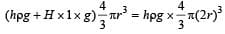This gives H = 7hρ

QUESTION: 3

### The angle of contact between pure water and pure glass, is 

Solution:

We know that angle of contact is the angle between the tangent to liquid surface at the point of contact and solid surface inside the liquid. In case of pure water and pure glass, the angle of contact is zero.

QUESTION: 4

A rectangular block of mass m and area of crosssection A floats in a liquid of density ρ. If it is given a small vertical displacement from equilibrium it undergoes  oscillation with a time period T. Then 

Solution:

Let the body be depressed by distance x from its equilibrium position. The extra upthrust created is xρAg which applies to whole body.
If a be acceleration created then,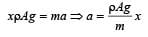Since, acceleration αx, so, it is equation of S.H.M.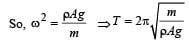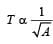QUESTION: 5

The wetability of a surface by a liquid depends primarily on [NEET 2013]

Solution:

Wetability of a surface by a liquid primarily depends on angle of contact between the surface and liquid.
If angle of contact is acute liquids wet the solid and vice-versa.

QUESTION: 6

A fluid is in streamline flow across a horizontal pipe of variable area of cross section. For this which of the following statements is correct? [NEET Kar. 2013]

Solution:

According to Bernoulli’s theorem,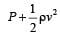= constant and Av = constant

If A is minimum, v is maximum, P is minimum.Use Code STAYHOME200 and get INR 200 additional OFF Use Coupon Code